# Flow Chart

A flowchart refers to the diagram that represent a process flow that enables in making decision making easy

## Flowchart Diagram representation

The following are the meaning of the diagrams to put at the back of you head when coming up with a flowchart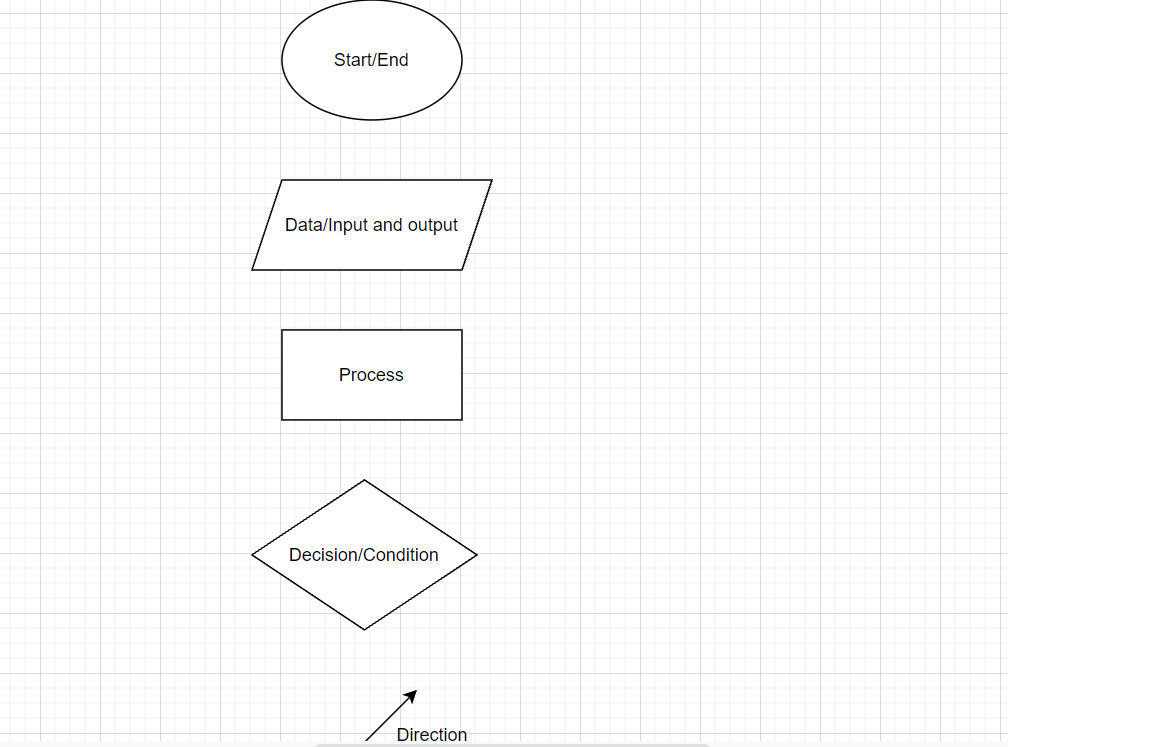## Example 1

The diagram below shows a flow chart for a program to calculate tax on an income.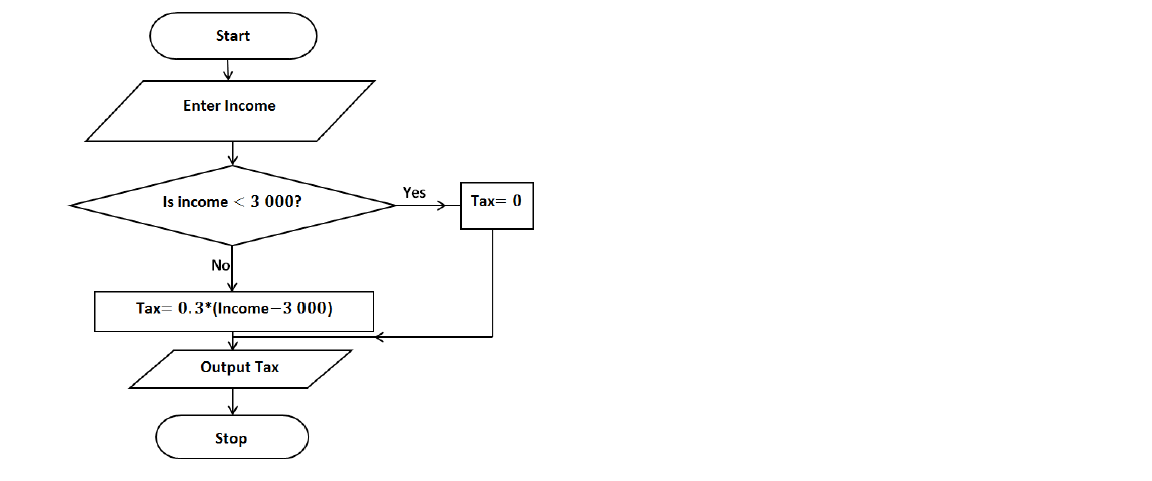Complete the below

$\begin{array}{|ll|}\hline Income& Tax\\ k2900& \\ k5000& \\ \hline\end{array}$

Solutions

In box 1 you’re just starting the program

In box 2 you enter the income then the program will do the calculation of tax

In box 2 the suggestion will be made by the program, If income is less than 3 000, the program will use the arrow where it says yes, tax will be zero and will be displayed in the Output Tax box.

Use the first income , is it less than 3 000?

Yes so the calculation will follow the yes route and tax will be zero

$\begin{array}{|ll|}\hline Income& Tax\\ k2900& 0\\ k5000& \\ \hline\end{array}$

Use the second income 5000 is it less than 3 000?

No so the calculation will follow the No route and tax will be calculated as follows

$Tax = 0.3 * \left(Income - 3000\right)$

Substitute into the formula, income = 5000.

$Tax = 0.3 * \left(5000 - 3000\right)$

$Tax = 0.3 * \left(2000\right)$

$Tax = 600$

Therefore below is the Completed diagram

$\begin{array}{|ll|}\hline Income& Tax\\ k2900& k0\\ k5000& k600\\ \hline\end{array}$

## Example 2

The diagram below is an incomplete program flow chart to calculate the area, A, of the triangle below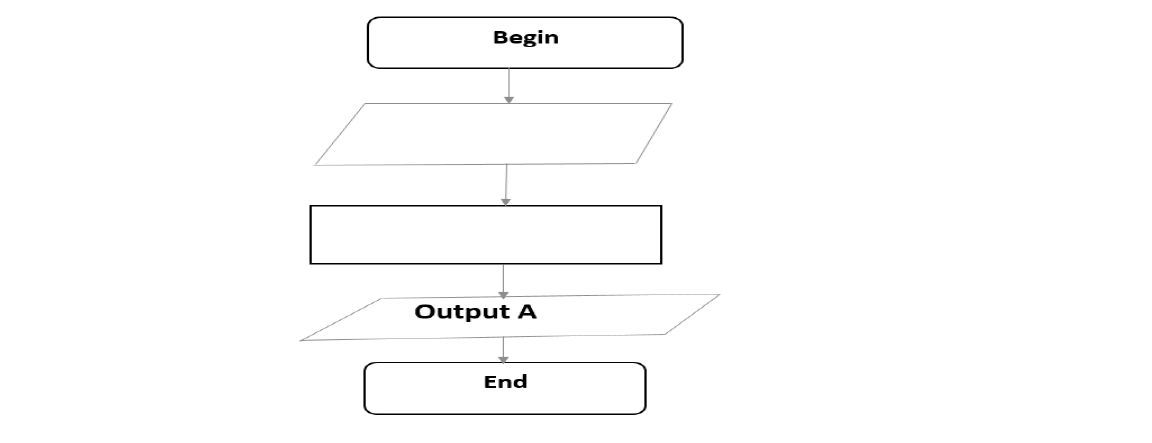Complete the flow chart below by writing appropriate statements in the blank symbols

Solutions

determine the formula to use for finding the area of a triangle. You have two sides k and ladjacent (attached) to the angle .

Use trigonometry formula $\frac{1}{2}×a×b×Sin\theta$

so when the computer program begin, you have to feed it with the values of k, l and $\theta$ and as indicated in the follow chart.

Enter the formula $\frac{1}{2}×a×b×Sin\theta$ In programming use * in place of $×$ . So the answer will be;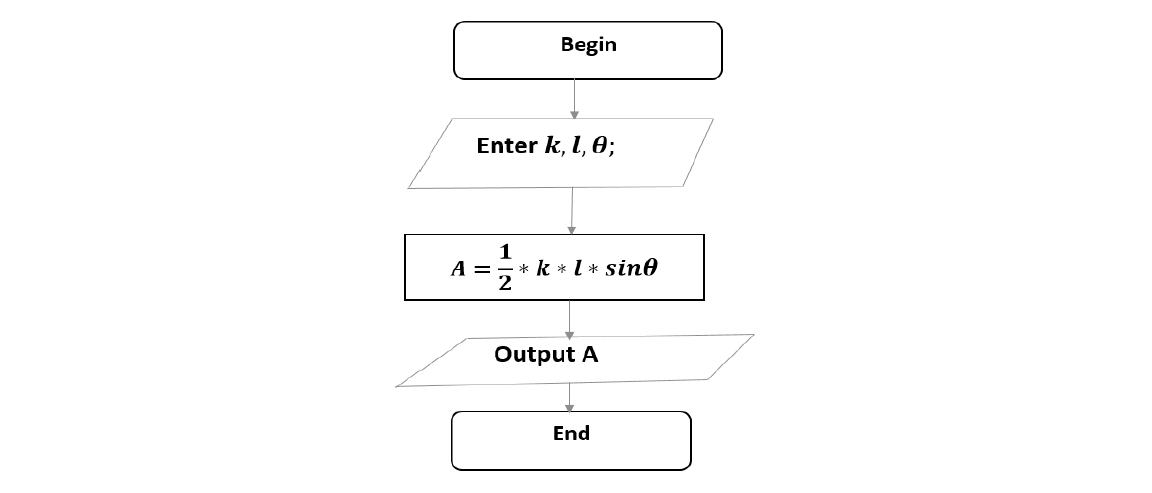## Draw flowchart from pseudocode

The programme below is given in form of a pseudocode.

Start

Then display "error message" and re-enter positive radius

Else enter slant height

If slant height < 0

Then display "error message" and re-enter positive slant height

Else Area = $\pi *r*\left(r + slant height\right)$

End if

Display Area

Stop

Draw the corresponding flowchart for the information given below

Solution: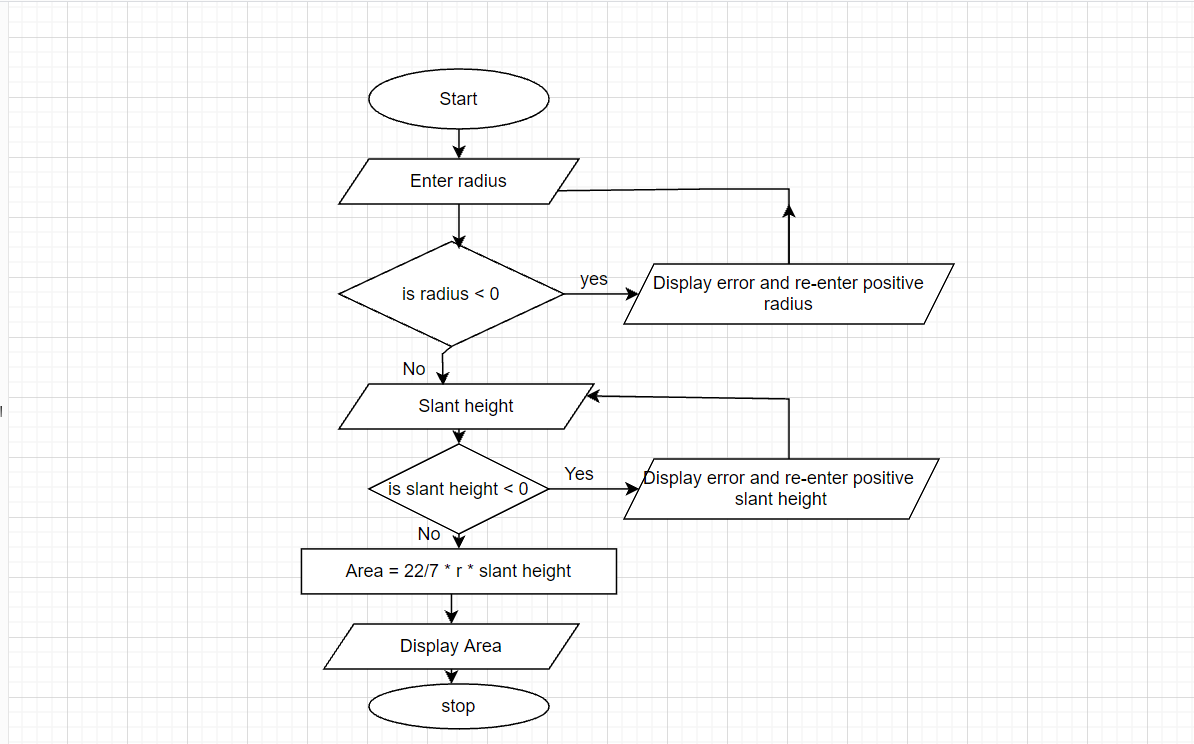## Write pseudocode from flowchart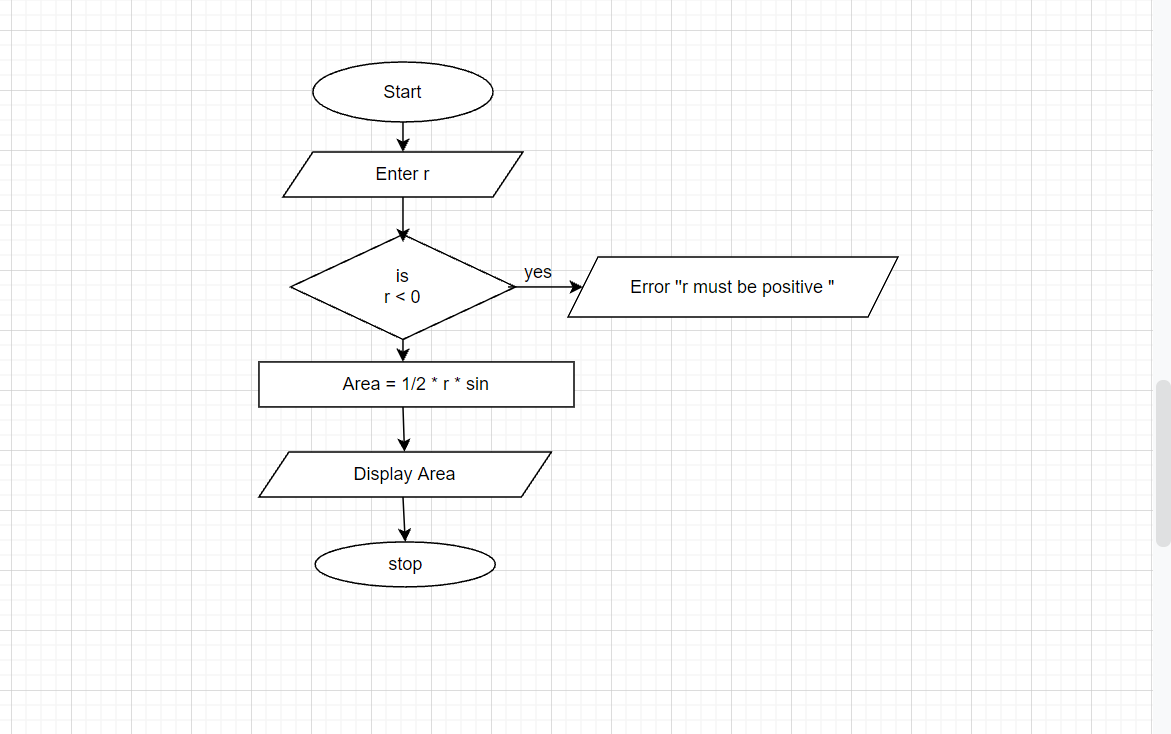Solution:

pseudocode

start

Enter r

if r < 0 , display error and enter positive r

if r > 0 then

let Area = 1/2 * square radius * sin

Then display Area and

Stop the program

## Flow Chart for Beginners PDF

Are you new to flow charts, pseudocode, and algorithms? If you're looking to understand these fundamental concepts used in programming and problem-solving, you've come to the right place. Our comprehensive guide is designed specifically for beginners, providing a step-by-step explanation of flow charts, pseudocode, and algorithms. To help you get started quickly, we're offering a PDF download of this guide. Download it now to gain a solid foundation in flow charts and enhance your problem-solving skills.

ECZ Flow Chart pdf